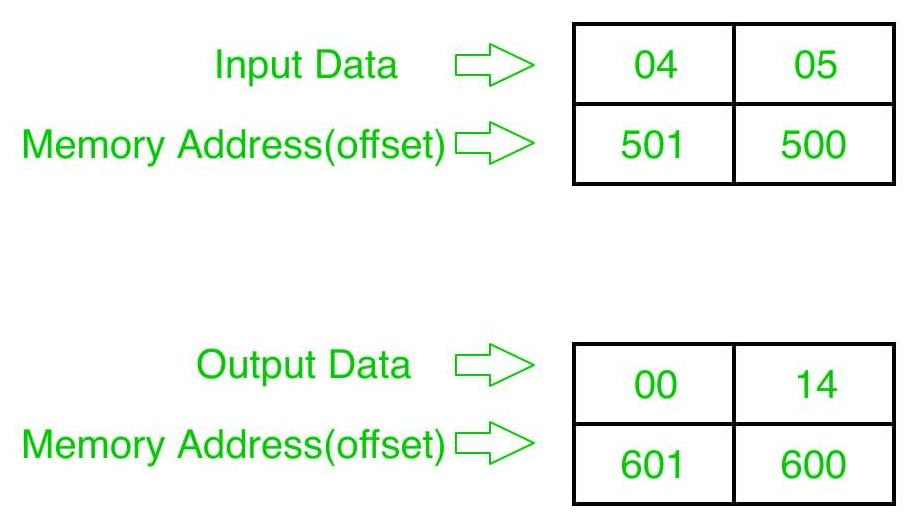GeeksforGeeks App
Open AppBrowser
Continue

# 8086 program to multiply two 8 bit numbers

Problem – Write a program in 8086 microprocessor to multiply two 8-bit numbers, where numbers are stored from offset 500 and store the result into offset 600.

Examples – Inputs and output are given in Hexadecimal representation.Algorithm –

1. Load data from offset 500 to register AL (first number)
2. Load data from offset 501 to register BL (second number)
3. Multiply them (AX=AL*BL)
4. Store the result (content of register AX) to offset 600
5. Stop

Program –

400MOV SI, 500SI=500
403MOV DI, 600DI=600
406MOV AL, [SI]AL<-[SI]
408INC SISI=SI+1
409MOV BL, [SI]BL<-[SI]
40BMUL BLAX=AL*BL
40DMOV [DI], AXAX->[DI]
40FHLTEND

Explanation –

1. MOV SI, 500 set 500 to SI
2. MOV DI, 600 set 600 to DI
3. MOV AL, [SI] load contents of offset SI to register AL
4. INC SI increase value of SI by 1
5. MOV BL, [SI] load contents of offset SI to register BL
6. MUL BL multiply contents of register AL and BL
7. MOV [DI], AX store the result (contents of register AX) to offset DI
8. HLT End.
My Personal Notes arrow_drop_up